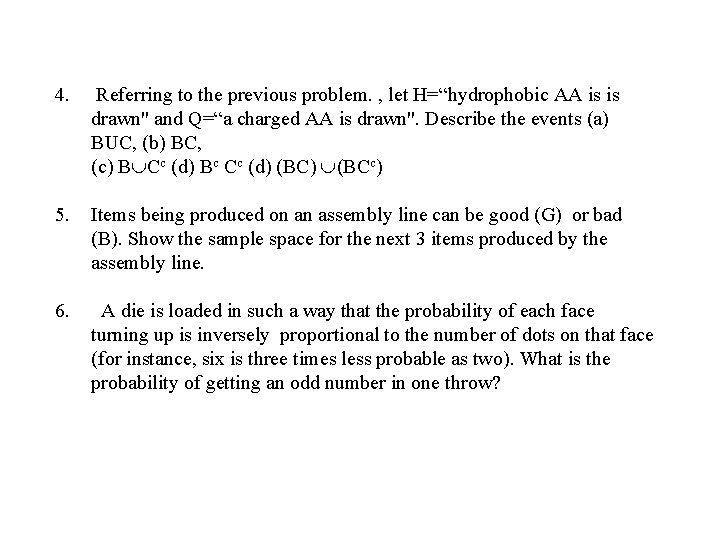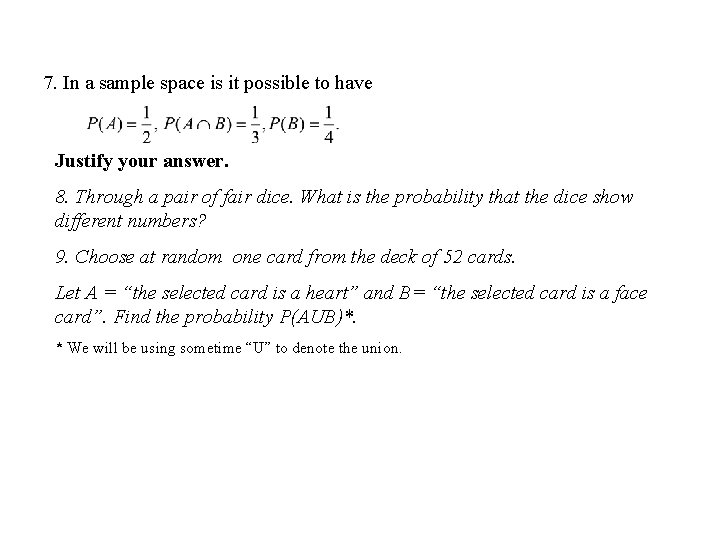# Home assignment 1 1 For what number of

• Slides: 5Home assignment 11. For what number of students n the probability that none of them have a birthday on the same day equals 0. 2? 2. A card is drawn at random from an ordinary deck of 52 playing cards. Describe the sample space if consideration of color (red or black) (a) is not, (b) is, taken in the account. Try the same if distinction between the “faces” and the “digits” (a) is not, (b) is, taken in the account. 3. Consider the set of 20 cards representing 20 amino acids (aa): {A, I, L, M, F, P, W, V, N, C, Q, G, S, T, Y, R, H, K, D, E}. It is known that some of the aa are usually charged while some others are neutral. Some of the aa are hydrophobic and some are hydrophilic. The card is drawn at random from this set. (a) Describe the events: C= “selected aa is typically charged”; N= “selected aa is neutral”; B = “aa is hydrophobic”; L= “aa is hydrophilic”. (b) Find their probabilities.4. Referring to the previous problem. , let H=“hydrophobic AA is is drawn" and Q=“a charged AA is drawn". Describe the events (a) BUC, (b) BC, (c) B Cc (d) Bc Cc (d) (BCc) 5. Items being produced on an assembly line can be good (G) or bad (B). Show the sample space for the next 3 items produced by the assembly line. 6. A die is loaded in such a way that the probability of each face turning up is inversely proportional to the number of dots on that face (for instance, six is three times less probable as two). What is the probability of getting an odd number in one throw?7. In a sample space is it possible to have Justify your answer. 8. Through a pair of fair dice. What is the probability that the dice show different numbers? 9. Choose at random one card from the deck of 52 cards. Let A = “the selected card is a heart” and B= “the selected card is a face card”. Find the probability P(AUB)*. * We will be using sometime “U” to denote the union.